CS 440/ECE 448
Margaret Fleck

## Convolutional neural nets

Convolutional neural nets are a specialized architecture designed to work well on image data (also apparently used somewhat for speech data). Images have two distinctive properties:

• They are large, e.g. 1000 by 1000 pixels.
• They have spatial coherence.

We'd like to be able to do some processing at high-resolution, to pick out features such as written text. But other objects (notably faces) can be recognized with only poor resolution. So we'd like to use different amounts of resolution at different stages of processing.

The large size of each layer makes it infeasible to connect units to every unit in the previous layer. Full interconnection can be done for artificially small (e.g. 32x32) input images. For larger images, this will create too many weights to train effectively with available training data. For physical networks (e.g. the human brain), there is also a direct hardware cost for each connection.

In a CNN, each unit reads input only from a local region of the preceding layer: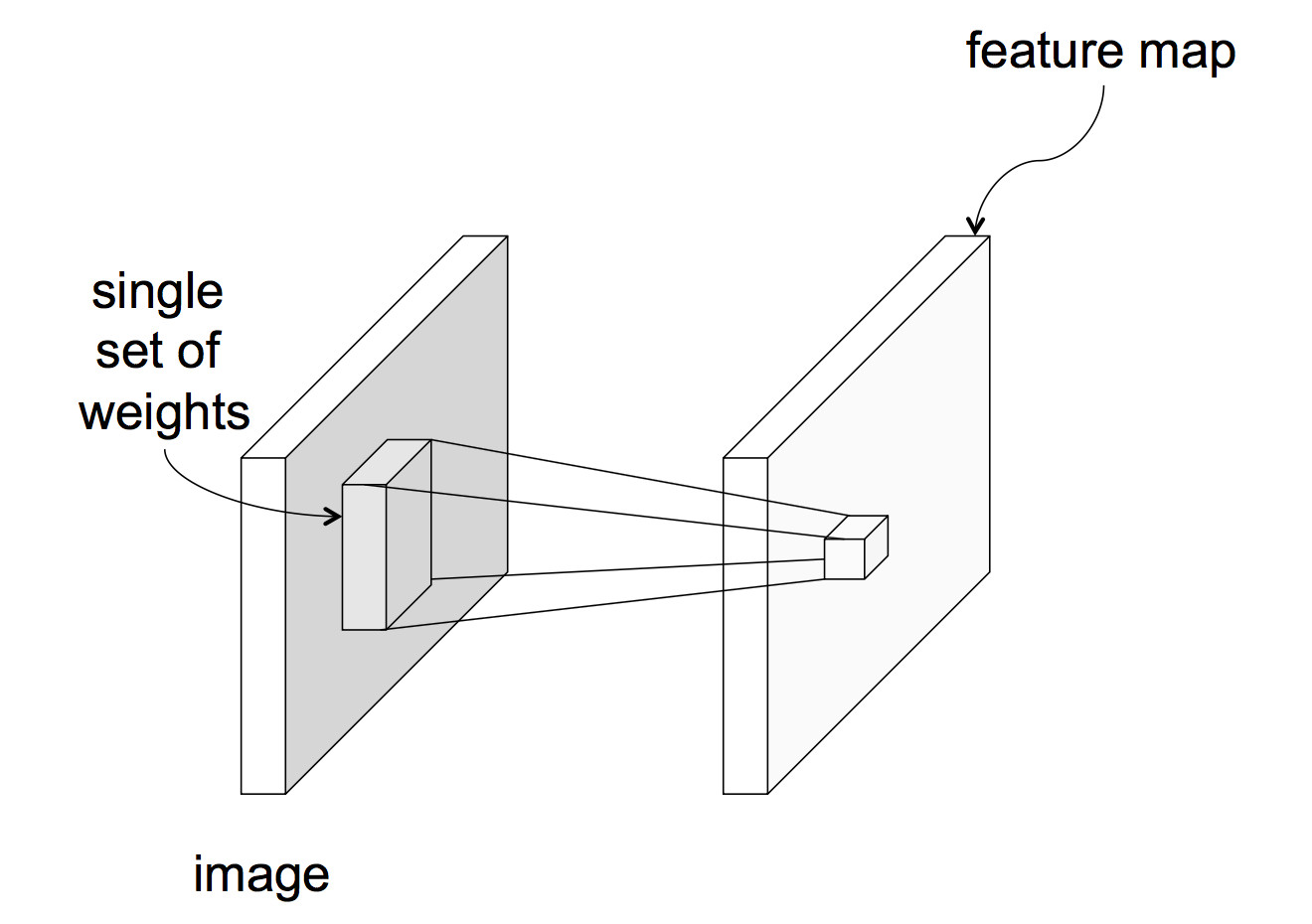from Lana Lazebnik Fall 2017

This means that each unit computes a weighted sum of the values in that local region. In signal processing, this is known as "convolution" and the set of weights is known as a "mask." For example, the following mask will locate sharp edges in the image.

              0   -4    0
-4   16   -4
0   -4    0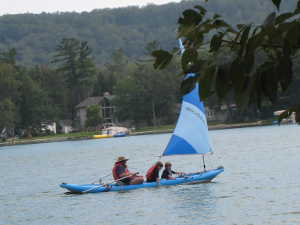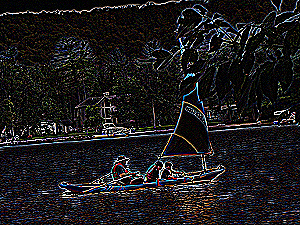The following mask detects horizontal edges but not vertical ones.

               2    4     8    4    2
0    0     0    0    0
-2   -4    -8   -4   -2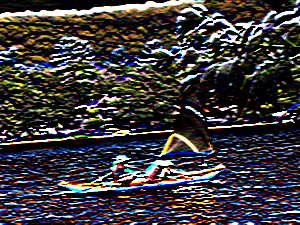These examples were made with gimp (select filters, then generic).

Normally, all units in the same layer would share a common set of weights and bias terms, called "parameter sharing". This reduces the number of parameters we need to train. However, parameter sharing may worsen performance if different regions in the input images are expected to have different properties, e.g. the object of interest is always centered. (This might be true in a security application where people were coming through a door.) So there are also neural nets in which separate parameters are trained for each unit in a convolutional layer.

## Convolutional layer

The above picture assumes that each layer of the network has only one value at each (x,y) position. This is typically not the case. An input image often has three values (red, green, blue) at each pixel. Going from the input to the first hidden layer, one might imagine that a number of different convolution masks would be useful to apply, each picking out a different type of feature. So, in reality, each network layer has a significant thickness, i.e. a number of different values at each (x,y) location.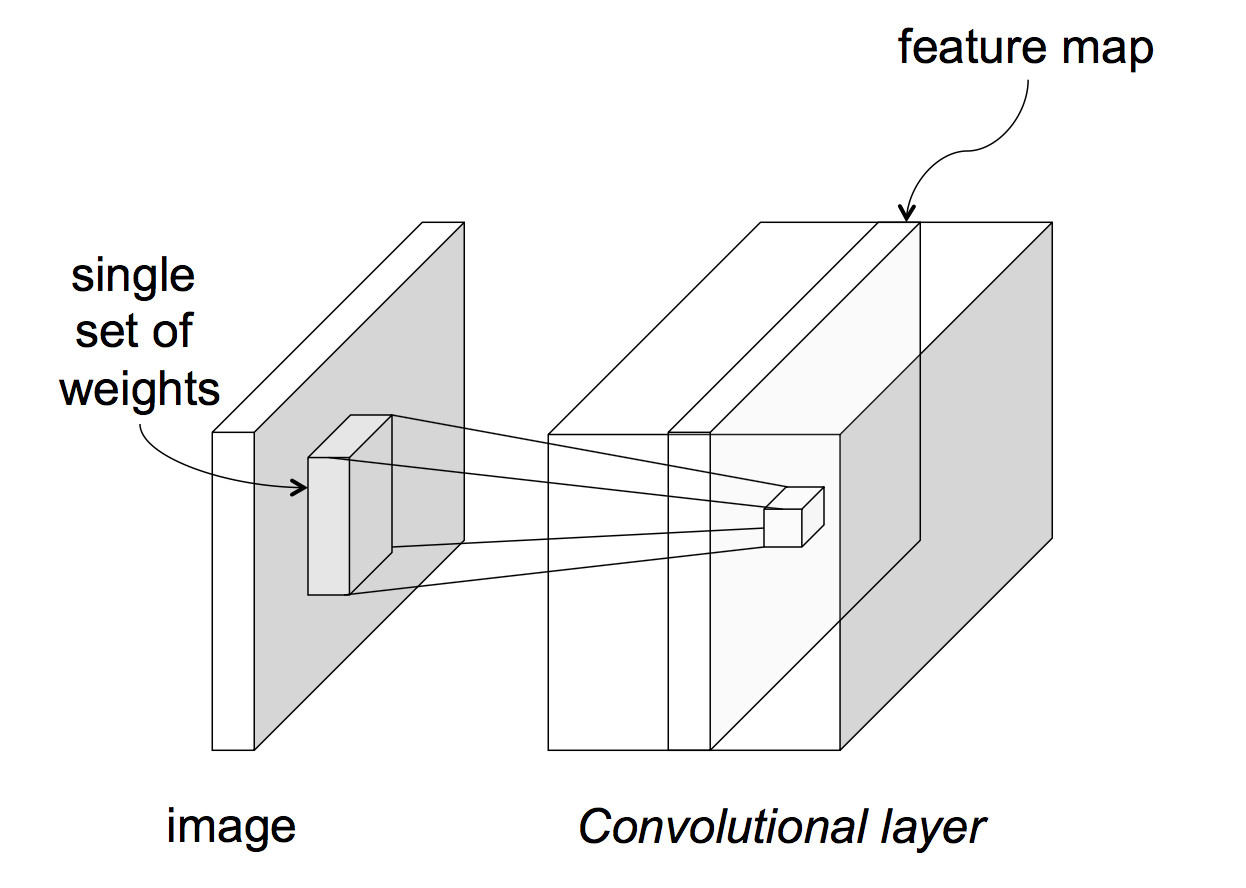from Lana Lazebnik Fall 2017

Click on the image below to see an animation from Andrej Karpathy shows how one layer of processing might work:

• blue: a 7x7 input image (3 color values at each location)
• red: the weights for two classifier units
• green: the outputs of the two units

In this example, each unit is producing values only at every third input location. So the output layer is a 3x3 image, with has two values at each (x,y) position.

Two useful bits of jargon:

• "stride" = how many pixels we shift mask sideways between output units
• "depth" = how many stacked features in each level

If we're processing color images, the initial input would have depth 3. But the depth might get significantly larger if we are extracting several different types of features, e.g. edges in a variety of orientations.

## Pooling

A third type of neural net layer reduces the size of the data, by producting an output value only for every kth input value in each dimension. This is called a "pooling" layer. The output values may be either selected input values, or the average over a group of inputs, or the maximum over a group of inputs.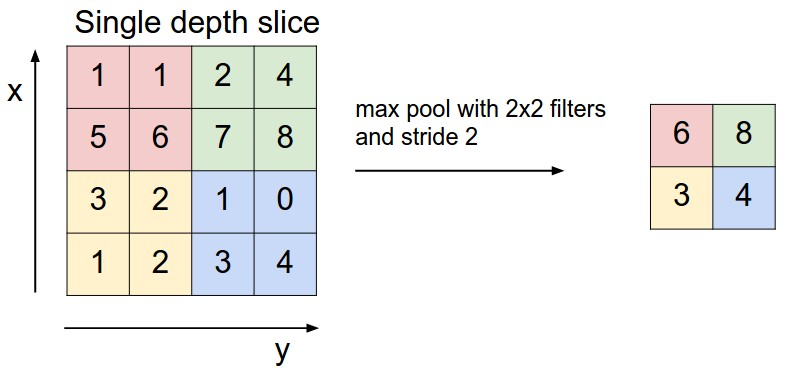from Andrej Karpathy

This kind of reduction in size ("downsampling") is especially sensible when data values are changing only slowly across the image. For example, color often changes very slowly except at object boundaries, and the human visual system represents color at a much lower resolution than brightness.

A pooling layer that chooses the maximum value can be very useful when we wish to detect high-resolution features but don't care about their precise location. E.g. perhaps we want to report that we found a corner, together with its approximate location.

## Architecture

A complete CNN typically contains three types of layers

• convolutional
• pooling
• fully-connected

Convolutional layers would typically be found in the early parts of the network, where we're looking at large amounts of image data. Fully-connected layers would make final decisions towards the end of the process, when we've reduced the image data to a smallish set of high-level features.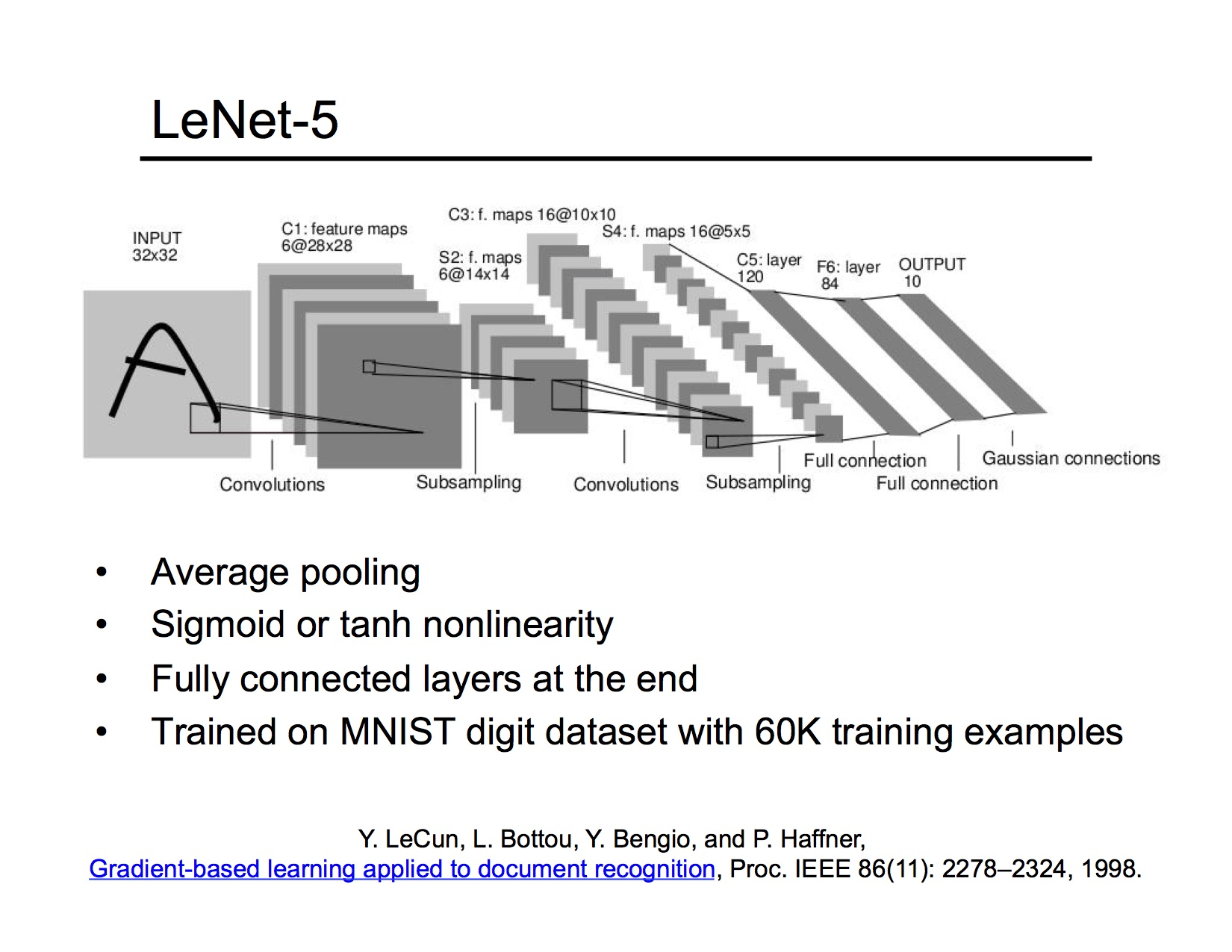The specific network in this picture is from 1998, when neural nets were just starting to be able to do something useful. Its input is very small, black and white, pictures of handwritten digits. The original application was reading zip codes for the post office.